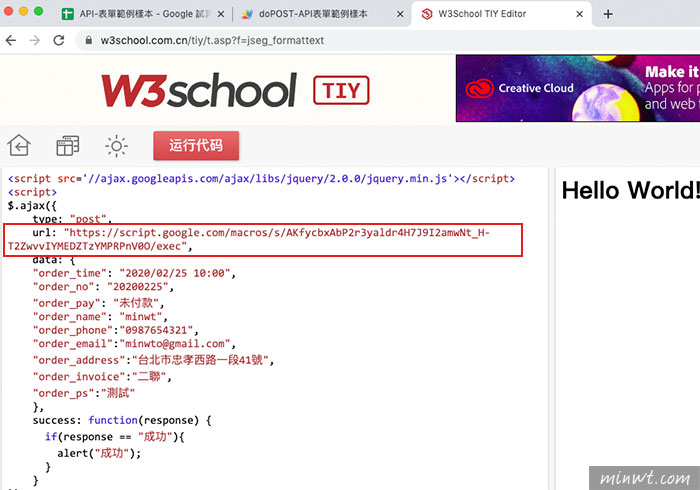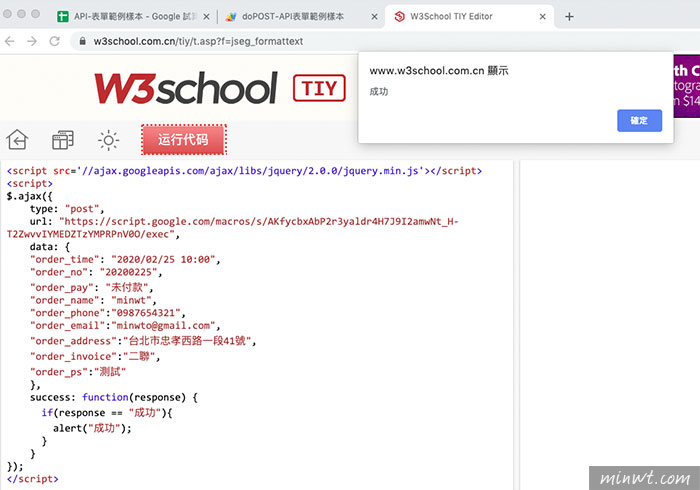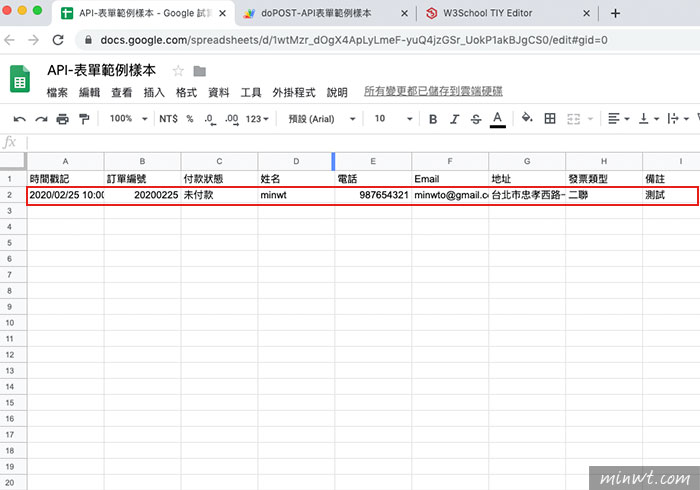1. 首頁
2. »
3. 軟體
4. »

2020/02/26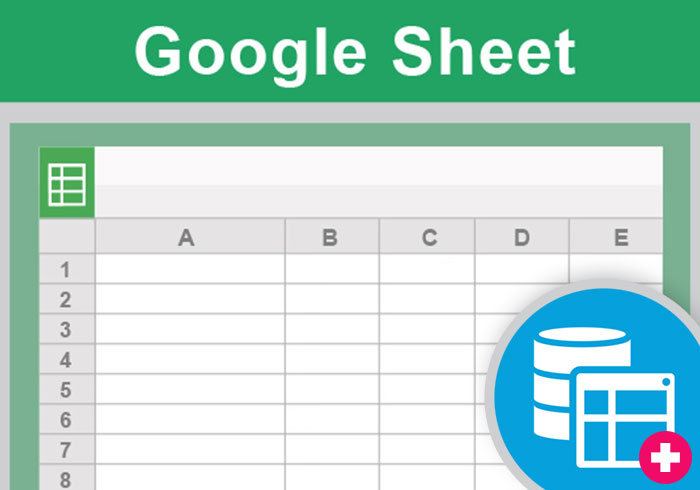Step1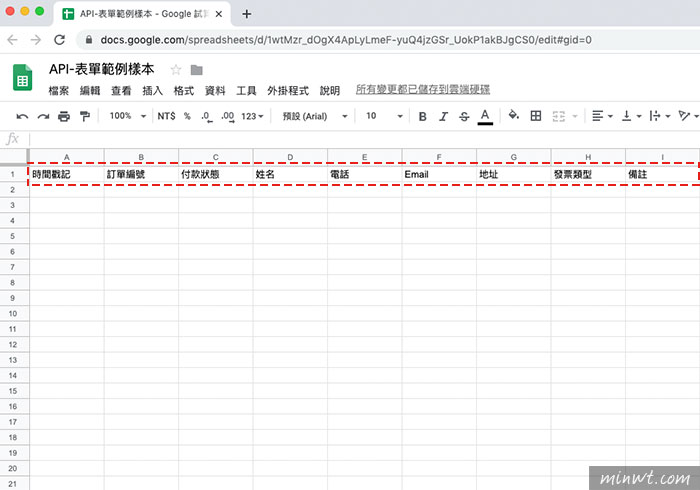Step2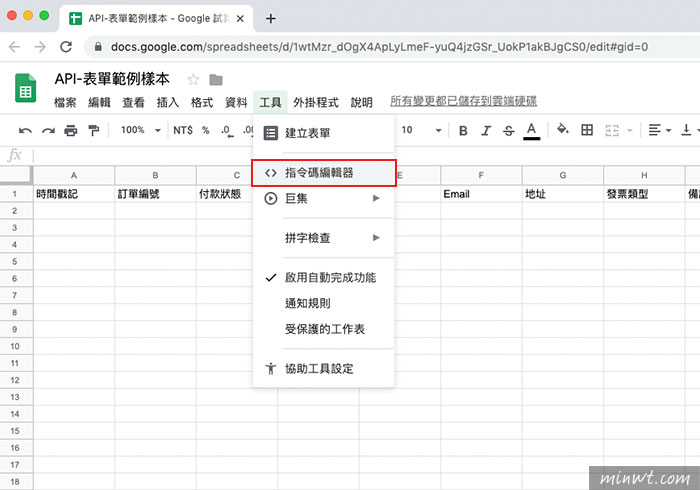Step3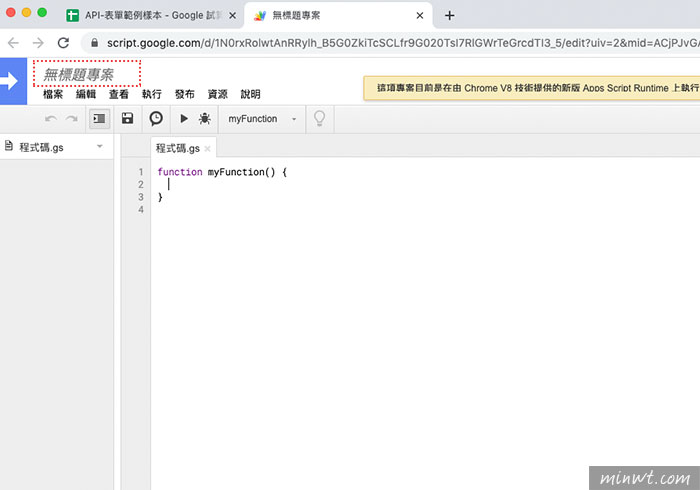Step4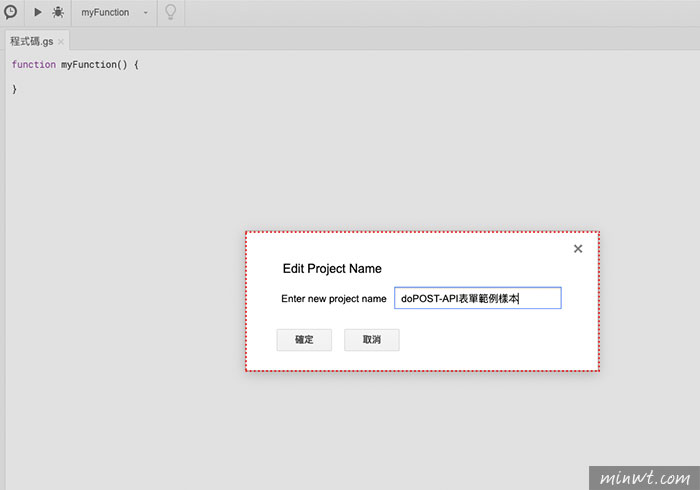Step5

```function doPost(e) {
//設定相應的欄位參數
var params = e.parameter; //這一個不要動
var time = params.order_time;
var no = params.order_no;
var pay = params.order_pay;
var name = params.order_name;
var phone = params.order_phone;
var email = params.order_email;
var invoice = params.order_invoice;
var ps = params.order_ps;
//設定sheet資訊
var LastRow = Sheet.getLastRow();
//資料寫入對應欄位中
Sheet.getRange(LastRow+1, 1).setValue(time);
Sheet.getRange(LastRow+1, 2).setValue(no);
Sheet.getRange(LastRow+1, 3).setValue(pay);
Sheet.getRange(LastRow+1, 4).setValue(name);
Sheet.getRange(LastRow+1, 5).setValue(phone);
Sheet.getRange(LastRow+1, 6).setValue(email);
Sheet.getRange(LastRow+1, 8).setValue(invoice);
Sheet.getRange(LastRow+1, 9).setValue(ps);
//當資料寫入完成後，回傳資訊
return ContentService.createTextOutput("成功");
}```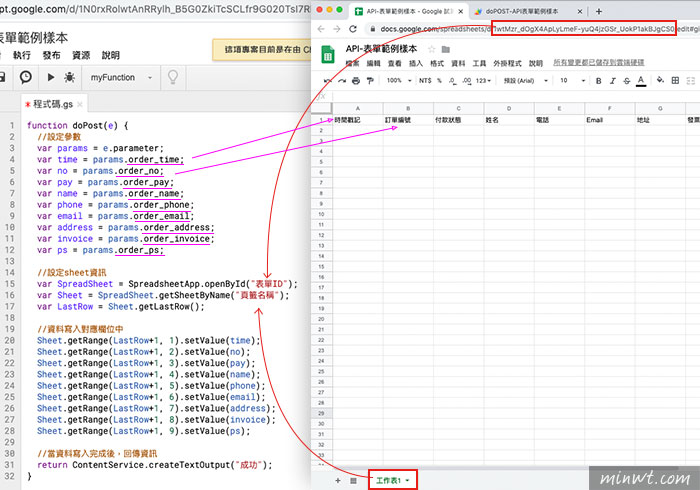1.doPost代表 API method 爲 post形式
2.利用 e.parameter，來分別設定每個試算表中的欄位
3.Sheet.getRange(LastRow+1, 1).setValue(order_time);這代表第一欄第一列為時間戳記，依此類推。

Step6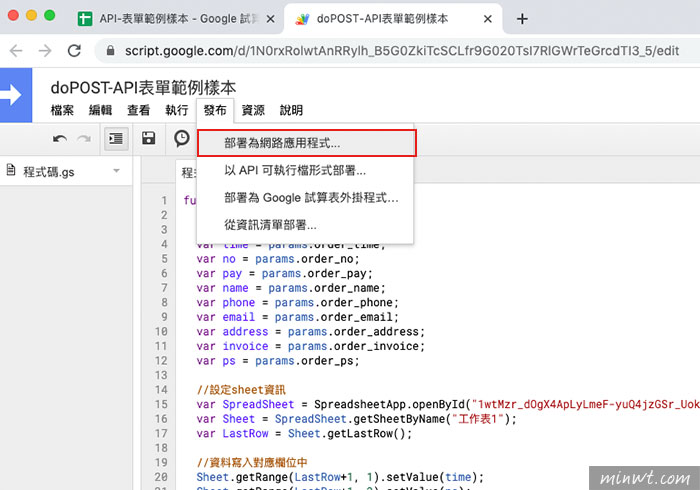Step7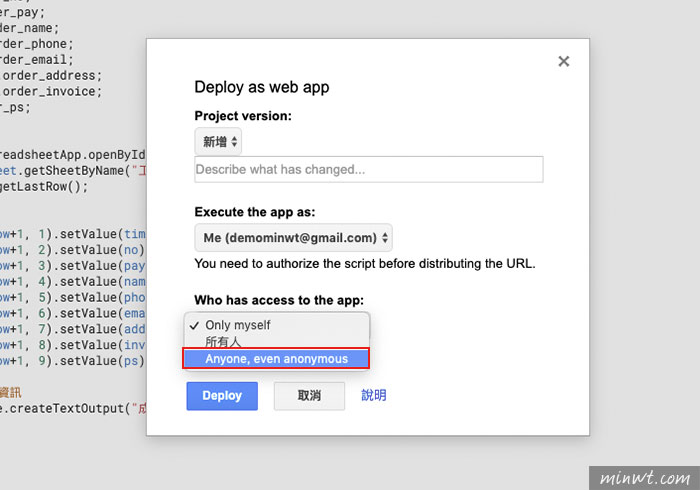Step8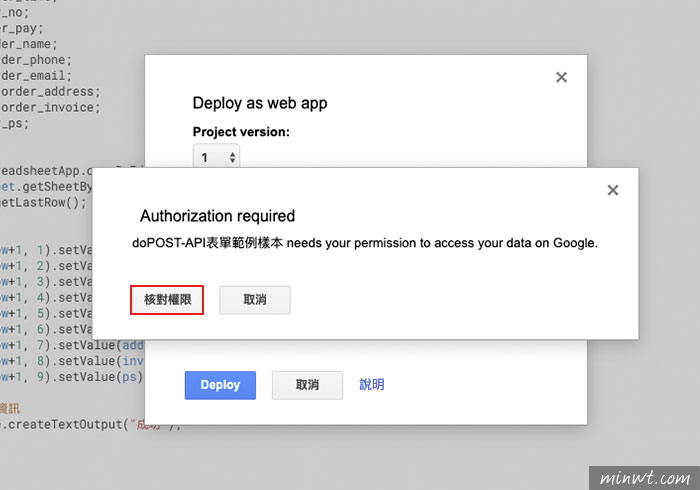Step9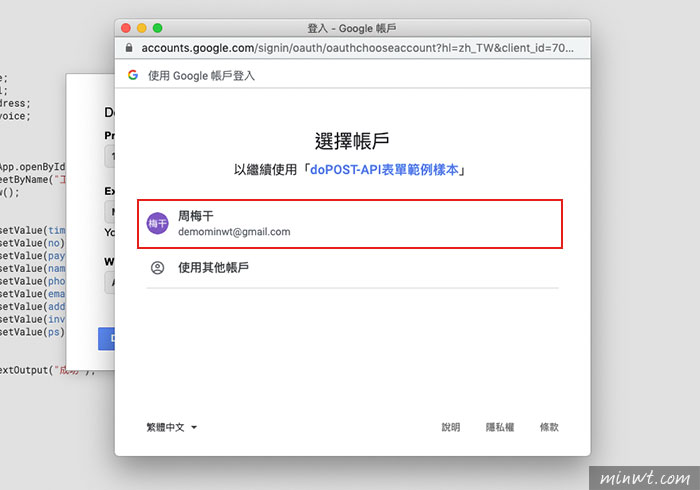Step10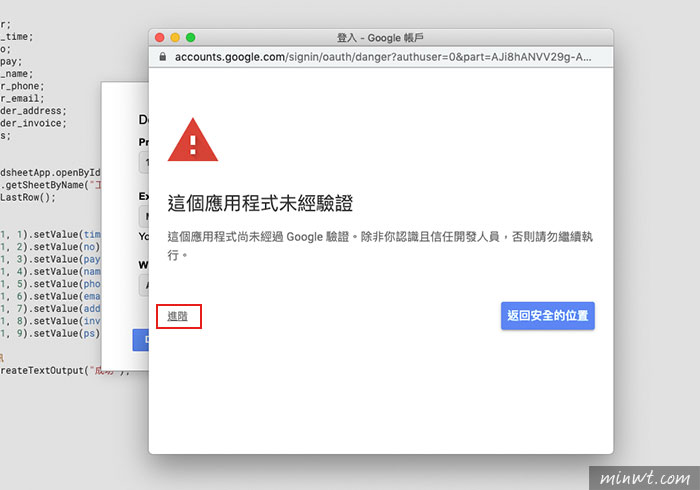Step11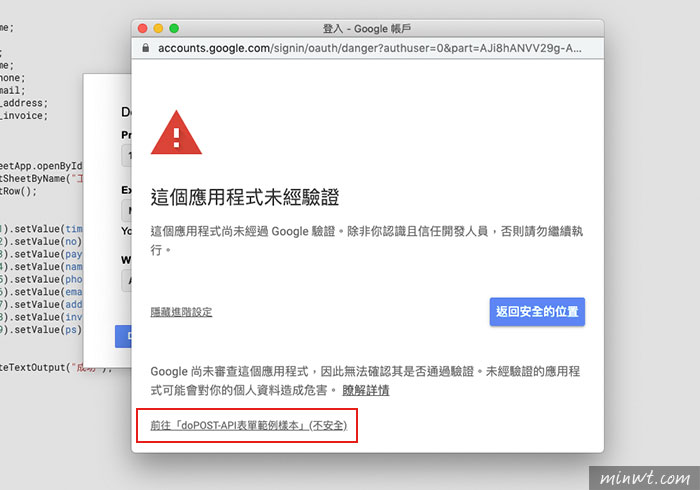Step12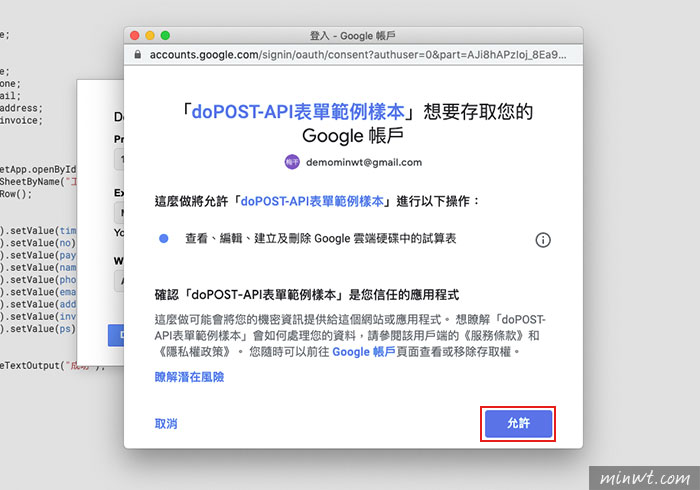Step13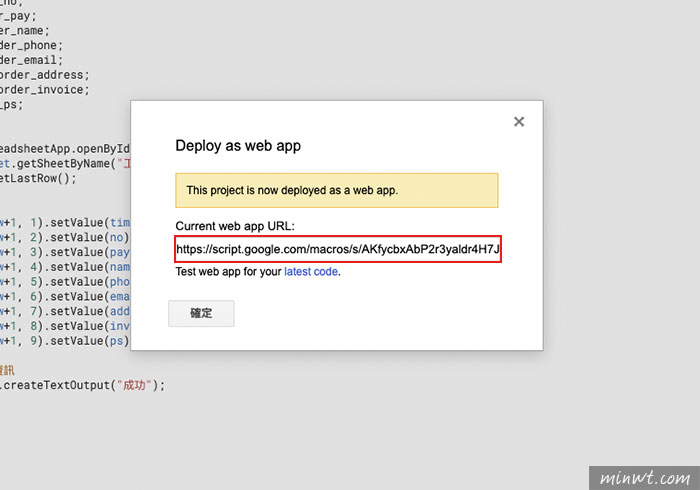Step14

Ajax call API 新增資料
```<script
src="//code.jquery.com/jquery-3.4.1.js"
integrity="sha256-WpOohJOqMqqyKL9FccASB9O0KwACQJpFTUBLTYOVvVU="
crossorigin="anonymous"></script>
<script>
\$.ajax({
type: "post",
url: "剛剛取得的 API URL",
data: {
"order_time": \$("input[name=time]").val(),
"order_no": \$("input[name=no]").val(),
"order_pay": \$("input[name=pay]").val(),
"order_name": \$("input[name=name]").val(),
"order_phone":\$("input[name=phone]").val(),
"order_email":\$("input[name=email]").val(),
"order_invoice":\$("select[name=invoice]").val(),
"order_ps":\$("input[name=ps]").val()
},
success: function(response) {
if(response == "成功"){